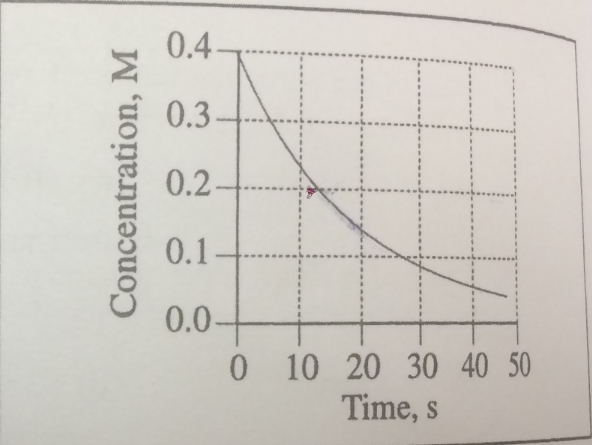# Problem: What is the initial rate of the reaction (in mol•L-1•s-1)  depicted by this graph?

###### FREE Expert Solution

Recall: Initial Rate

Picking the smallest change in concentration and time:

Δ[ ] = [ ]final – [ ]initial = 0.4 M – 0.3 M = 0.1 M

Δt = tfinal – tinitial = 5 s – 0 s = 5 s###### Problem Details

What is the initial rate of the reaction (in mol•L-1•s-1)  depicted by this graph?